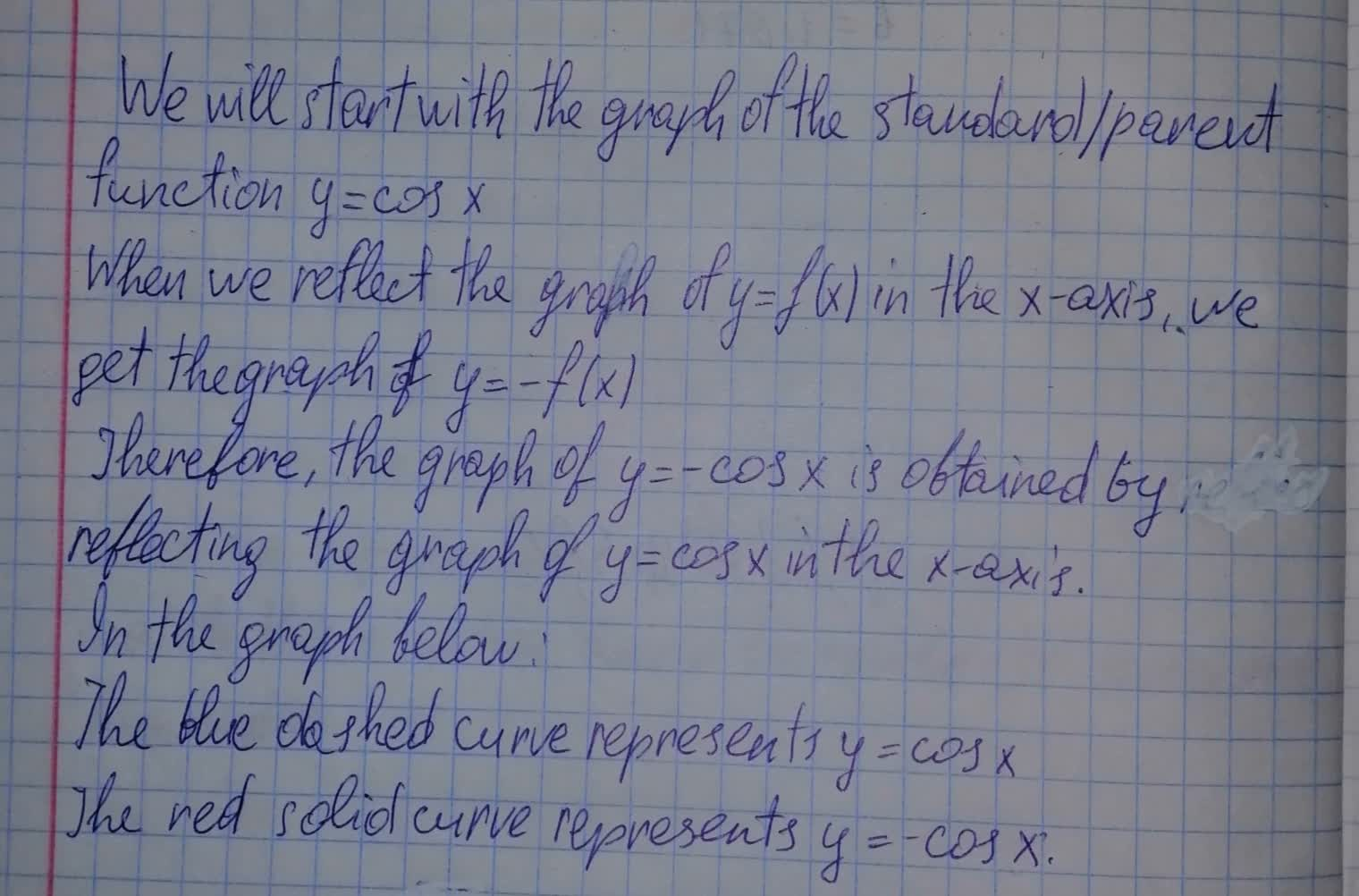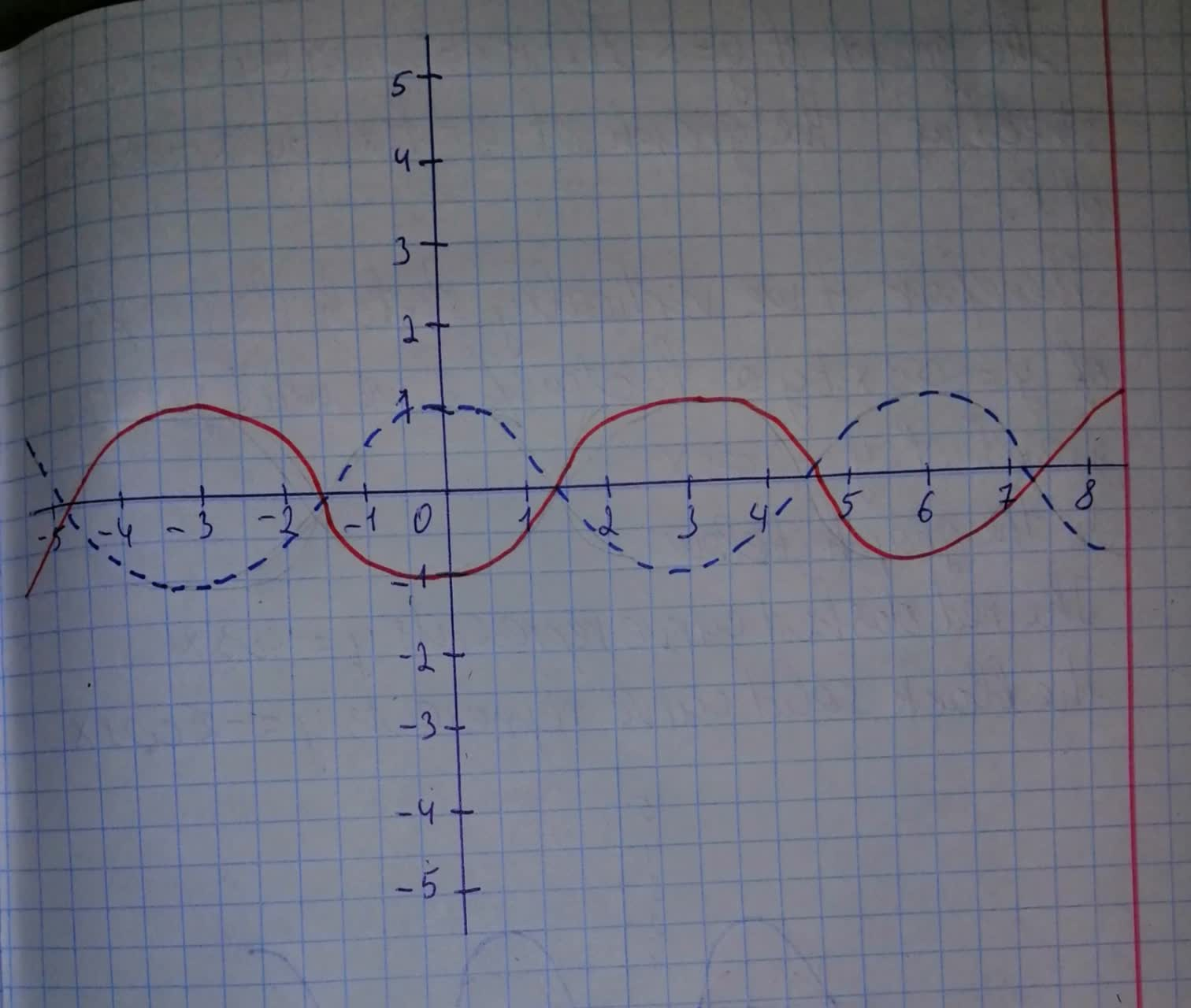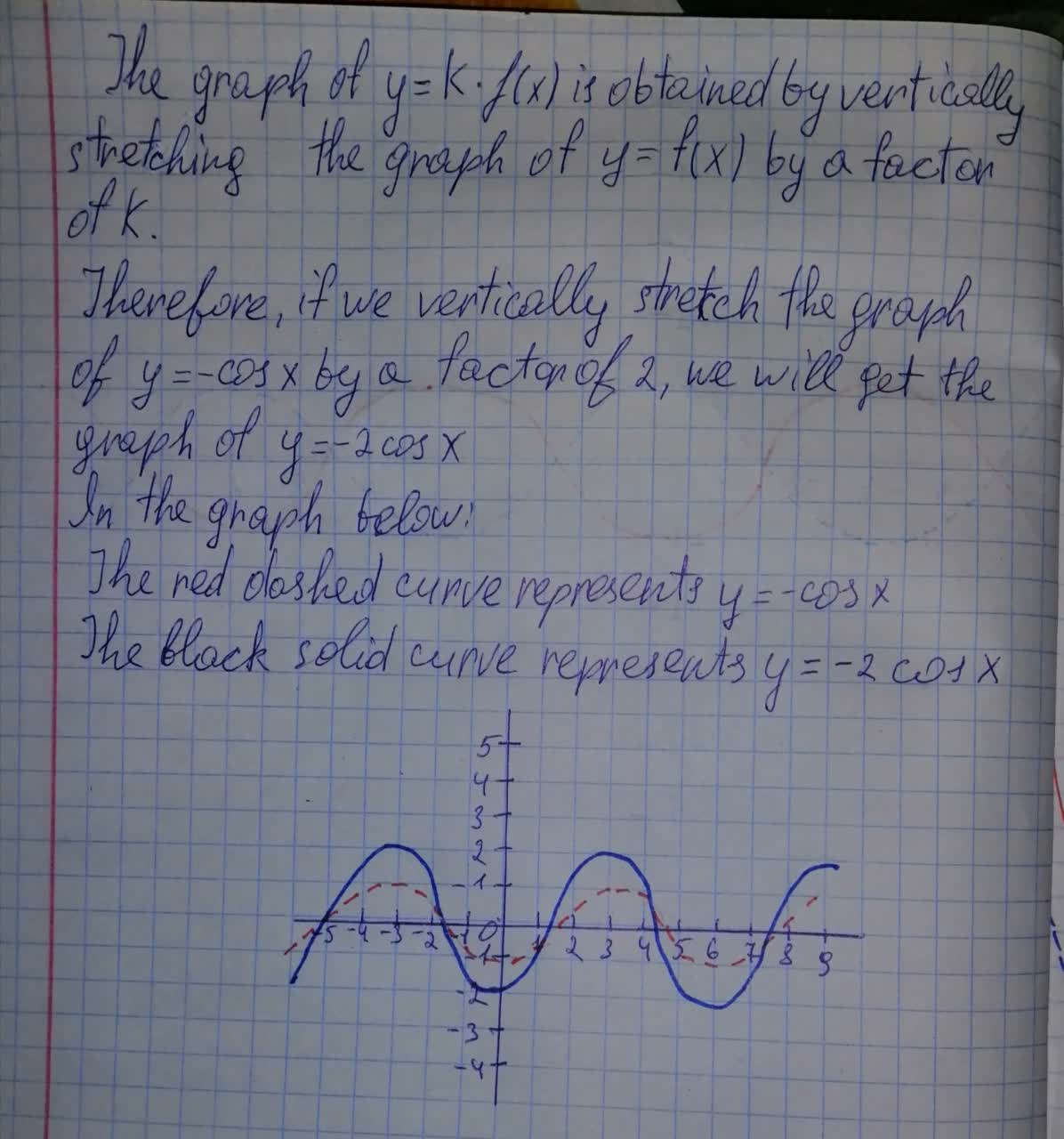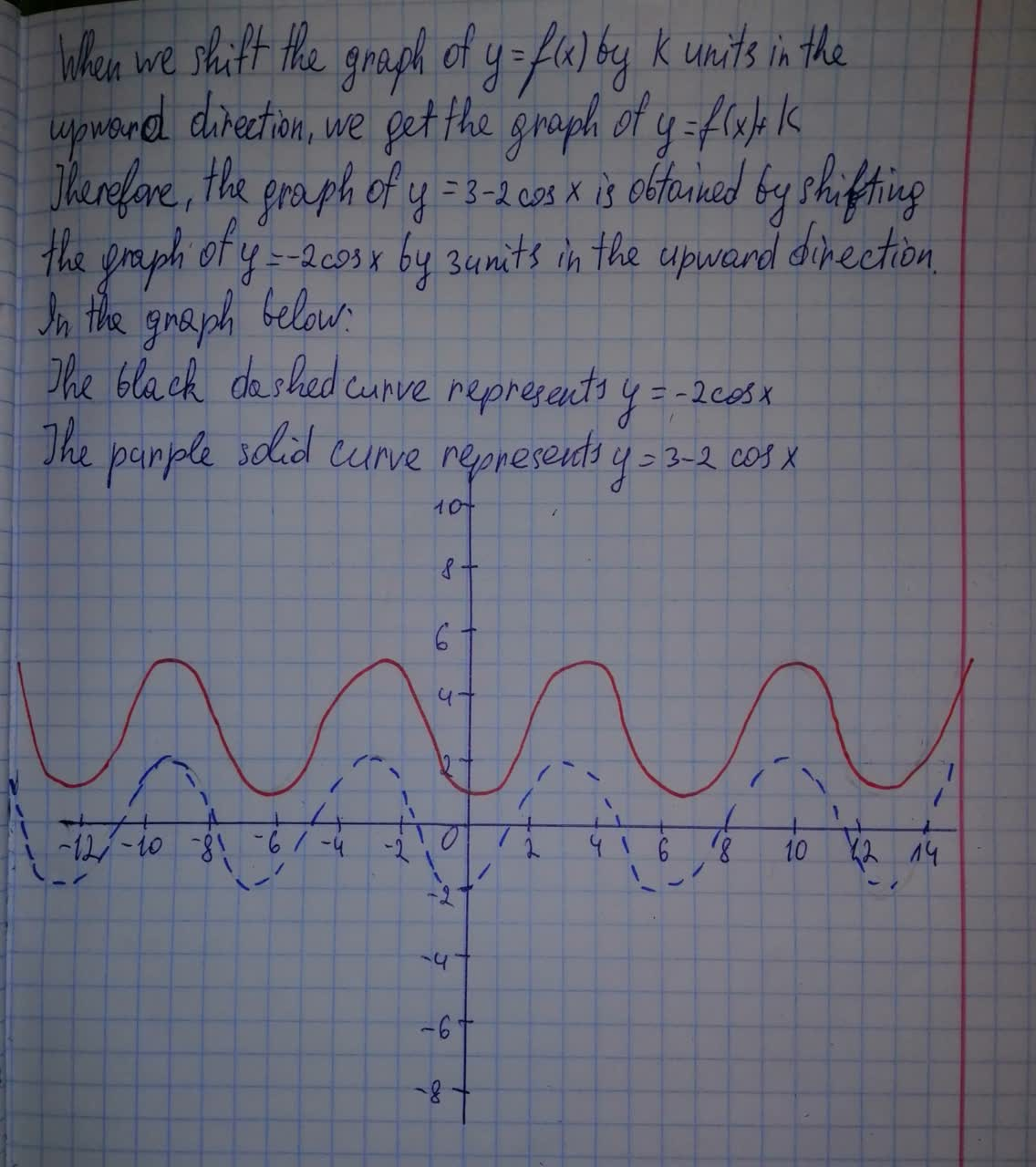# Graph the function by hand, not by plotting points, but by starting with the graph of one of the standard functions. y=3-2 \cos xAva-May Nelson 2021-07-13 Answered
Graph the function by hand, not by plotting points, but by starting with the graph of one of the standard functions, and then applying the appropriate transformations.
$y=3-2\mathrm{cos}x$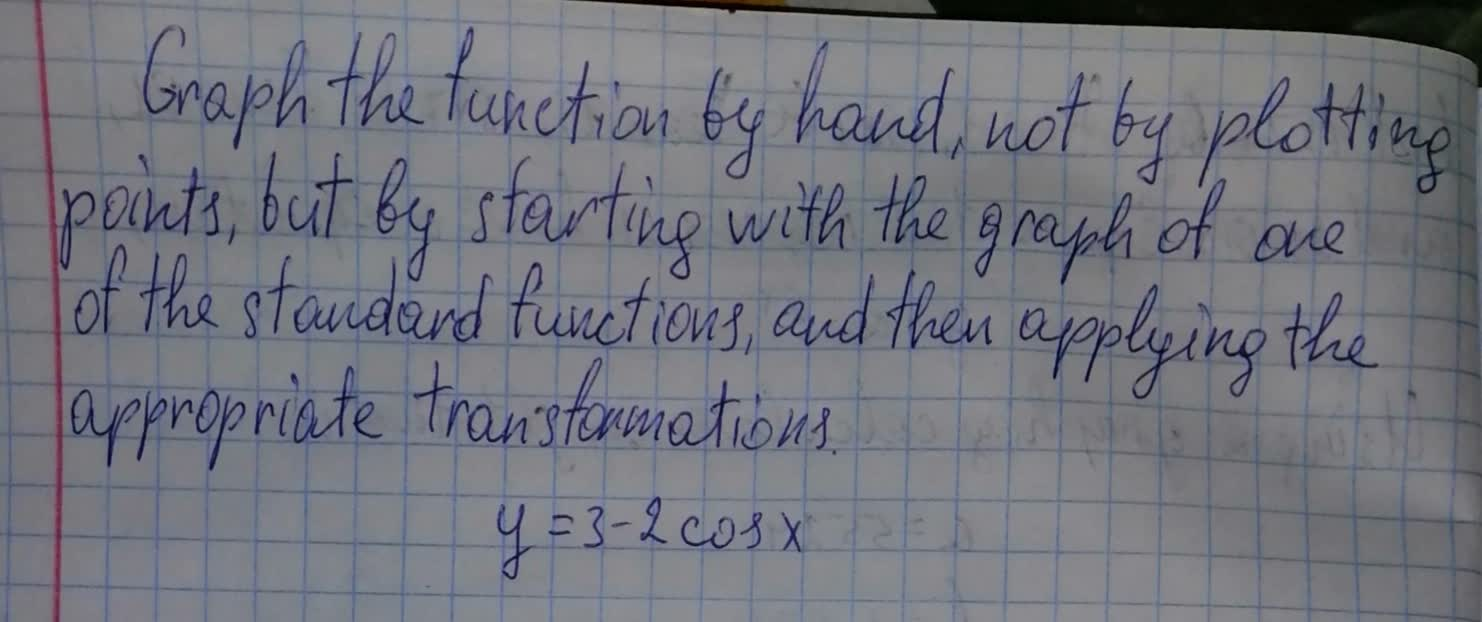You can still ask an expert for help

• Questions are typically answered in as fast as 30 minutes

Solve your problem for the price of one coffee

• Math expert for every subject
• Pay only if we can solve itBrittany Patton